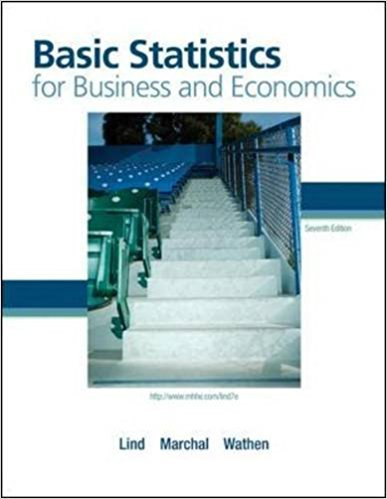×
Get Full Access to Basic Statistics For Business And Economics - 7 Edition - Chapter 6 - Problem 5e
Get Full Access to Basic Statistics For Business And Economics - 7 Edition - Chapter 6 - Problem 5e

×

# The information below is the number of daily emergencyISBN: 9780077384470 64

## Solution for problem 5E Chapter 6

Basic Statistics for Business and Economics | 7th Edition

• Textbook Solutions
• 2901 Step-by-step solutions solved by professors and subject experts
• Get 24/7 help from StudySoup virtual teaching assistantsBasic Statistics for Business and Economics | 7th Edition

4 5 1 381 Reviews
13
4
Problem 5E

Problem 5E

The information below is the number of daily emergency service calls made by the volunteer ambulance service of Walterboro, South Carolina, for the last 50 days. To explain, there were 22 days on which there were 2 emergency calls, and 9 days on which there were 3 emergency calls.

 Number of Calls Frequency 0 8 1 10 2 22 3 9 4 1 Total 50

a. Convert this information on the number of calls to a probability distribution.

b. Is this an example of a discrete or continuous probability distribution?

c. What is the mean number of emergency calls per day?

d. What is the standard deviation of the number of calls made daily?

Step-by-Step Solution:

Solution :

Step 1 of 4:

Given the number of daily emergency service calls.

Then the table is given below.

 Number of Calls Frequency 0 8 1 10 2 22 3 9 4 1 Total 50

Our goal is:

a). We need to convert the number of calls to a probability distribution.

b). We need to find this example is discrete or continuous probability distribution.

c). We need to find the mean.

d). We need to find the standard deviation.

a). Now we have to convert the number of calls to a probability distribution.

Then the table is given below.

 Number of Calls (X) Frequency P(X)XP(X)0 8= 0.16 00.16 = 0 0.4624 1 10=0.2 10.2 = 0.2 0.098 2 22=0.44 20.44 = 0.88 0.0396 3 9=0.18 30.18 =0.54 0.3042 4 1=0.02 40.02 =0.08 0.1058 Total 501.7= 1.01

From the above table mean is 1.7 and the variance is 1.01.

Step 2 of 3

Step 3 of 3

##### ISBN: 9780077384470

This textbook survival guide was created for the textbook: Basic Statistics for Business and Economics , edition: 7. This full solution covers the following key subjects: calls, emergency, days, were, information. This expansive textbook survival guide covers 6 chapters, and 325 solutions. Since the solution to 5E from 6 chapter was answered, more than 5100 students have viewed the full step-by-step answer. Basic Statistics for Business and Economics was written by and is associated to the ISBN: 9780077384470. The full step-by-step solution to problem: 5E from chapter: 6 was answered by , our top Business solution expert on 08/23/17, 08:36AM. The answer to “The information below is the number of daily emergency service calls made by the volunteer ambulance service of Walterboro, South Carolina, for the last 50 days. To explain, there were 22 days on which there were 2 emergency calls, and 9 days on which there were 3 emergency calls.Number of CallsFrequency081102223941Total50a. Convert this information on the number of calls to a probability distribution.________________b. Is this an example of a discrete or continuous probability distribution?________________c. What is the mean number of emergency calls per day?________________d. What is the standard deviation of the number of calls made daily?” is broken down into a number of easy to follow steps, and 96 words.

Unlock Textbook Solution